# Matrices and arrays in MATLAB

The basics of MATLAB were discussed in the previous module. It covered topics on interface of MATLAB software and the basic operations of MATLAB along the help and documentation features. Furthermore, this article discusses the matrices and array part of MATLAB.

Array is an arrangement of elements in a way that all the elements in this arrangements are similar in terms of size, data type and are arranged one after other continously in the memory (Barnett & Tongoa, 2008). Matrix (matrices in plural form) is a multidimensional array. In the present module the different operations on array, their use and methods to use array, traversing in array and  functions available for array in MATLAB are focused .The present article discusses about the basics of array and the basic operations that can be performed on an array.

## Array creation

Enter elements in a row and separate the elements either by a comma or a space in order to create arrays as shown below.Image 1: Enter elements in row matrices

The image above presents a row vector. In order to create a column matrix, separate each number by semicolon as shown below. Furthermore, separate rows by semicolon to create matrix with multiple rows as shown in image 3.Image 2 a): Creating column matrixImage 2 b): Creating matrix with multiple rows

Use functions like zeros, ones or rand to create a matrix. Use function ‘zero( )’ to create a matrix with all zeros, ‘ones( )’  creates matrix with all elements with one and finally ‘rand( )’  is used to create a matrix with random numbers. Pass the size of the matrix as arguments in these functions as shown in image below.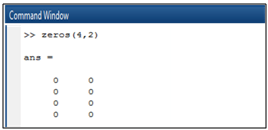Image 3 (a) Matrices created using function zero ( )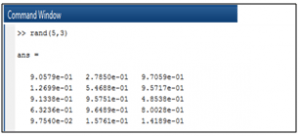Image 3 (b) Matrices created using function rand ( )

## Operations applied on Matrices

Different operations can be applied on matrices, using a single arithmetic operator like transpose of matrices, inverse of matrices or matrices multiplication both row wise and column wise, element wise multiplication and many more.  Single arithmetic operations are as follows:

1. Trigonometric functions: To perform trigonometric operations, pass the elements or the variables  as arguments in the trigonometric functions as shown below.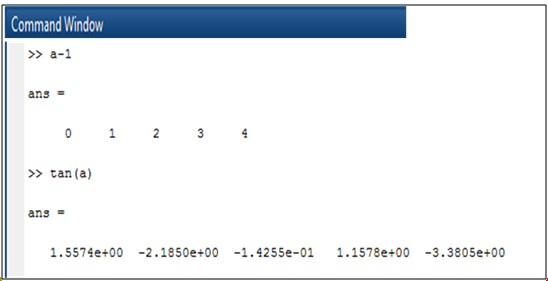Image 4: Use of trigonometric function on matrices

1. Transpose matrix: Transposing a matrix means interchanging the rows and columns. Use single quote (‘) to transpose a matrix  and perform matrix multiplication as follows as shown below.Image 5 a) Transpose of a Matrix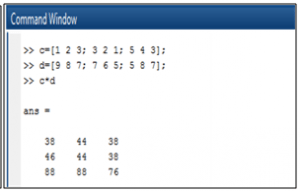Image 5 b)Matrix multiplication

1. Multiplication: To perform element wise multiplication instead of matrix multiplication, use ‘.*’ as shown below.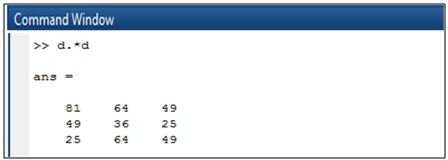Image 6: Element wise multiplication in matrices

1. Inverse of matrix: To find inverse of a matrix, use function ‘inv()’ (image below)Image 7: Inverse of matrices

1. Concatenate arrays: Two arrays are joined using square brackets as shown below.This is called horizontal concatenation wherein the number of rows in the concatenation arrays should be equal. Similarly, use a semicolon for vertical concatenation . In vertical concatenation, number of columns in two concatenating arrays should be equal as shown below.Image 8 a): Row WiseImage 8 b): Column Wise Concatenation

1. Complex and imaginary numbers: Complex numbers have area part and an imaginary part that is square root of -1 as stated in the image below. Furthermore, MATLAB uses i or j to represent an imaginary part in a number as shown in image 9 b).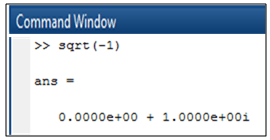Image 9 a): Matrices of complex numbersImage 9 b): Imaginary numbers

### Soumya Srivastava

Research Analyst at Project Guru
Soumya has completed her MBA in HR from National Institute of Technology (NIT), Kurukshetra and is Bachelor in Information Technology. She loves numbers, their patterns and the infinity nature possessed by them. When it comes to computers, she has interest in memory allocation and virtual memory. In her spare time she likes to read sun signs and personality traits. She believes in hard work and considers smart work to be incomplete without hard work.

#### Latest posts by Soumya Srivastava (see all)We are looking for candidates who have completed their master's degree or Ph.D. Click here to know more about our vacancies.